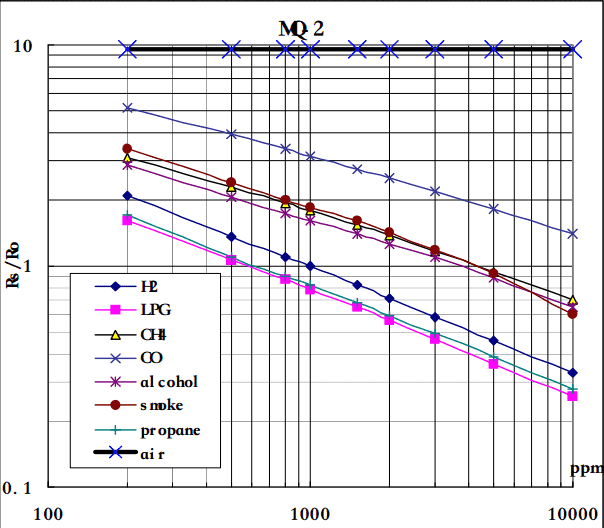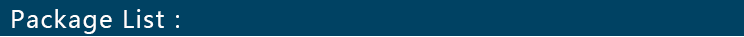Description

The Puzzle - Gas Sensor(MQ2) module is useful for gas leakage detecting(in home and industry). It can detect LPG, i-butane, methane, alcohol, Hydrogen, smoke and so on. Based on its fast response time. measurements can be taken as soon as possible. Also the sensitivity can be adjusted by the potentiometer.

Features
• Fast response
• Covers LPG, i-butane, methane, alcohol, Hydrogen etc
• Useful for gas leakage detecting
• Puzzle connector compatible

Note

Hot-swapping the grove may lead to IC burnout, please turn off the power of main board before swapping puzzle.

## Application Ideas

• Gas leakage detection.
• Toys.

## Hardware Overview

This is an Analog output sensor. It needs to be connected to any one Analog socket in Puzzle Base Shield. The examples used in this tutorial make uses of A0 analog pin. Connect this module to the A0 port of Base Shield.

It is possible to connect the Grove module to Arduino directly by using jumper wires, please refer to the connection in the table below:

ArduinoGas Sensor
5VVCC
GNDGND
NCNC
Analog A0SIG

The output voltage from the Gas sensor increases when the concentration of gas increases. Sensitivity can be adjusted by rotating the potentiometer. Please note that the best preheat time for the sensor is above 24 hours. For detail information about the MQ-2 sensor, please refer the data-sheet provided in Documents section.

### Gas Detection : Basic Example

In this example, the sensor is connected to A0 pin. The voltage read from the sensor is displayed. This value can be used as a threshold to detect any increase/decrease in gas concentration.

``````void setup() {
Serial.begin(9600);
}

void loop() {
float sensor_volt;
float sensorValue;

sensor_volt = sensorValue/1024*5.0;

Serial.print("sensor_volt = ");
Serial.print(sensor_volt);
Serial.println("V");
delay(1000);
}
``````

### Measurement : Approximation

These examples demonstrate ways to know the approximate concentration of Gas. As per the data-sheet of the MQx sensors, these equations are tested for standard conditions and are not calibrated. It may vary based on change in temperature or humidity.

• Keep the Gas Sensor in clean air environment. Upload the program below.
``````void setup() {
Serial.begin(9600);
}

void loop() {
float sensor_volt;
float RS_air; //  Get the value of RS via in a clear air
float R0;  // Get the value of R0 via in H2
float sensorValue;

/*--- Get a average data by testing 100 times ---*/
for(int x = 0 ; x < 100 ; x++)
{
}
sensorValue = sensorValue/100.0;
/*-----------------------------------------------*/

sensor_volt = sensorValue/1024*5.0;
RS_air = (5.0-sensor_volt)/sensor_volt; // omit *RL
R0 = RS_air/9.8; // The ratio of RS/R0 is 9.8 in a clear air from Graph (Found using WebPlotDigitizer)

Serial.print("sensor_volt = ");
Serial.print(sensor_volt);
Serial.println("V");

Serial.print("R0 = ");
Serial.println(R0);
delay(1000);

}
``````
• Then, open the serial monitor of Arduino IDE. Write down the value of R0 and this will be used in the next program. Please write down the R0 after the reading stabilizes.

Replace the R0 below with value of R0 tested above . Expose the sensor to any one of the gas listed above.

``````void setup() {
Serial.begin(9600);
}

void loop() {

float sensor_volt;
float RS_gas; // Get value of RS in a GAS
float ratio; // Get ratio RS_GAS/RS_air
sensor_volt=(float)sensorValue/1024*5.0;
RS_gas = (5.0-sensor_volt)/sensor_volt; // omit *RL

/*-Replace the name "R0" with the value of R0 in the demo of First Test -*/
ratio = RS_gas/R0;  // ratio = RS/R0
/*-----------------------------------------------------------------------*/

Serial.print("sensor_volt = ");
Serial.println(sensor_volt);
Serial.print("RS_ratio = ");
Serial.println(RS_gas);
Serial.print("Rs/R0 = ");
Serial.println(ratio);

Serial.print("\n\n");

delay(1000);

}
``````

Now, we can get the concentration of gas from the figure below.According to the graph, we can see that the minimum concentration we can test is 100ppm and the maximum is 10000ppm, in a other word, we can get a concentration of gas between 0.01% and 1%. However, we can’t provide a formula because the relation between ratio and concentration is nonlinear.

Documents

Puzzle - Gas Sensor MQ-2 datasheet: MQ-2.pdf• 1pcs Duinopeak Puzzle - MQ2 Gas Sensor